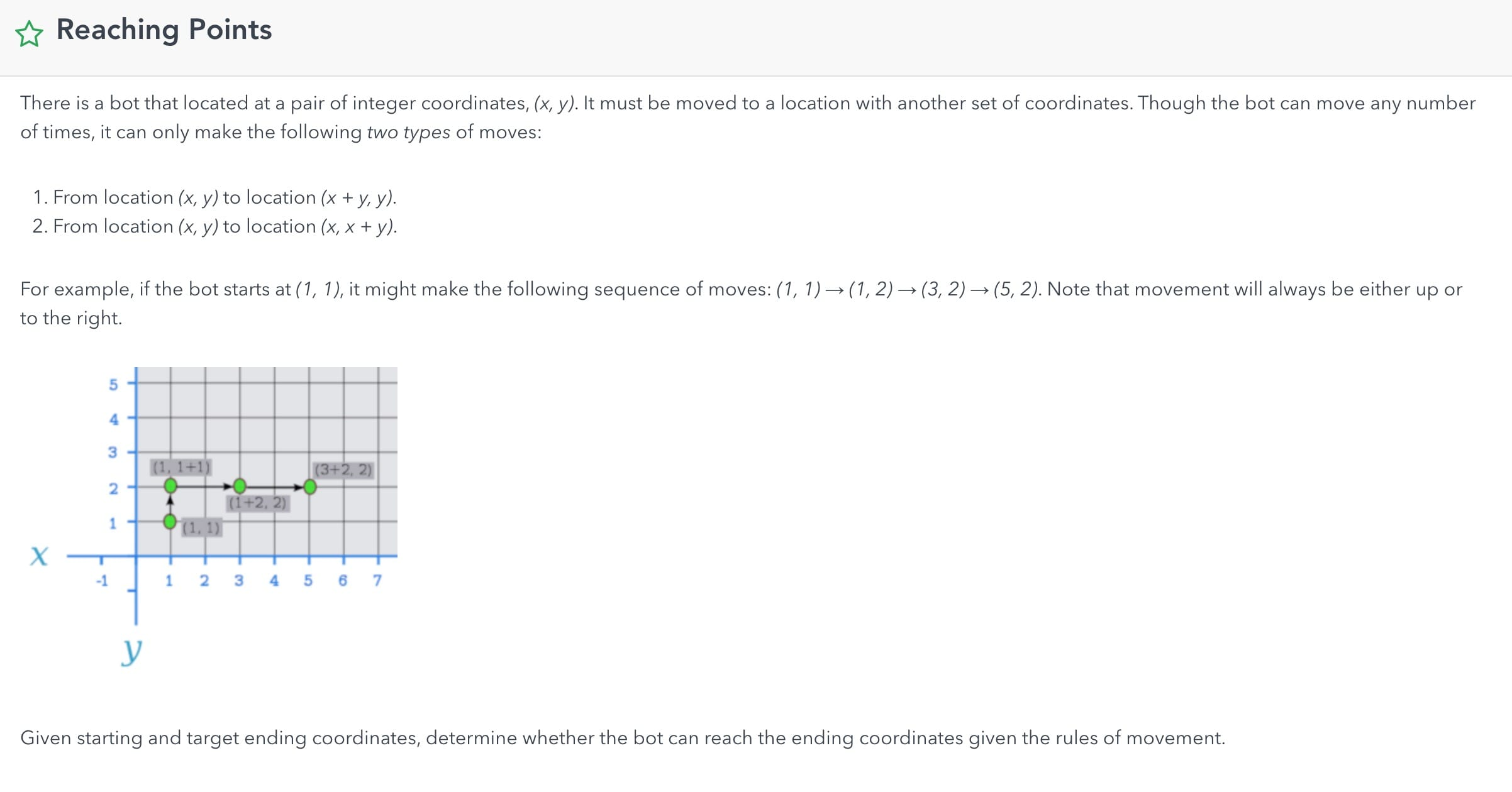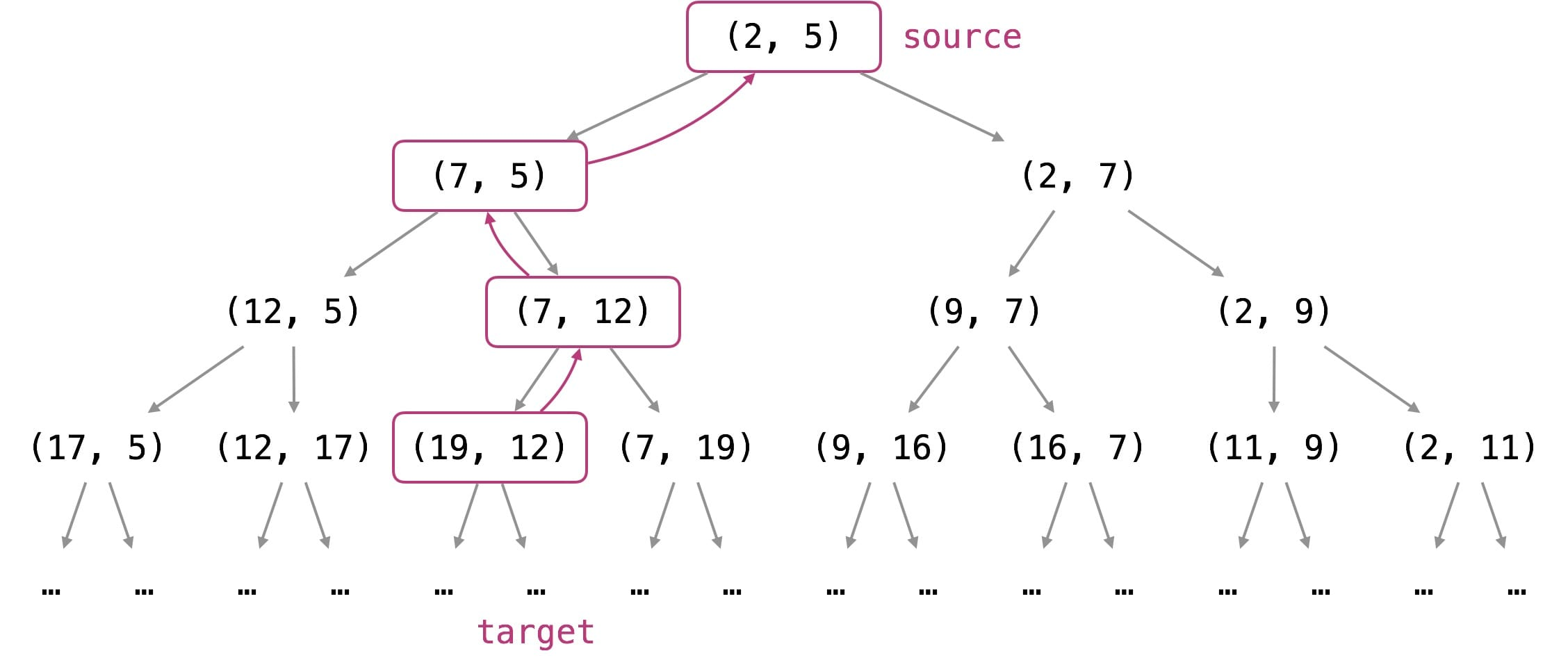# 780. Reaching Points

Reference: LeetCode
Difficulty: Hard

## Problem

A move consists of taking a point (x, y) and transforming it to either (x, x+y) or (x+y, y).

Given a starting point (sx, sy) and a target point (tx, ty), return True if and only if a sequence of moves exists to transform the point (sx, sy) to (tx, ty). Otherwise, return False.Note: sx, sy, tx, ty will all be integers in the range [1, 10^9].

Example:

## Analysis

### Brute-Force

Time: $O(2^{tx + ty})$, a loose bound found by considering every move as (x, y) -> (x + 1, y) or (x, y) -> (x, y + 1).
Space: $O(tx + ty)$, the size of call stack.

### Work Backwards (Naive Variant)

From LeetCode solution:Time: $O(\max{(tx, ty)})$. If say ty = 1, we could do subtraction for tx times.
Space: $O(1)$

### Work Backwards (Modulo Variant)

Time: $O(\log{\max{(tx, ty)}})$
Space: $O(1)$

CommentJunhao Wang
a software engineering cat